# Normalization

There are various normalizations in statistics

Name Formula Use
Standard score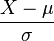$\frac{X - \mu}{\sigma}$ Normalizing errors when population parameters are known. Works well for populations that are normally distributed
Student’s t-statistic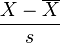$\frac{X - \overline{X}}{s}$ Normalizing residuals when population parameters are unknown (estimated).
Studentized residual$\frac{\hat \epsilon_i}{\hat \sigma_i} = \frac{X_i - \hat \mu_i}{\hat \sigma_i}$ Normalizing residuals when parameters are estimated, particularly across different data points in regression analysis.
Standardized moment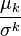$\frac{\mu_k}{\sigma^k}$ Normalizing moments, using the standard deviation$\sigma$ as a measure of scale.
Coefficient of
variation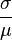$\frac{\sigma}{\mu}$ Normalizing dispersion, using the mean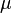$\mu$ as a measure of scale, particularly for positive distribution such as theexponential distribution and Poisson distribution.
Feature scaling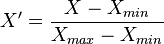$X' = \frac{X - X_{min}}{X_{max}-X_{min}}$ Feature scaling used to bring all values into the range [0,1]. This is also called unity-based normalization. This can be generalized to restrict the range of values in the dataset between any arbitrary points$a$ and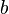$b$ using$X' = a + \frac{\left(X-X_{min}\right)\left(b-a\right)}{X_{max} - X_{min}}$.

Advertisements

This site uses Akismet to reduce spam. Learn how your comment data is processed.